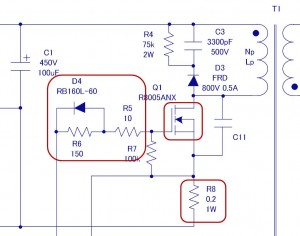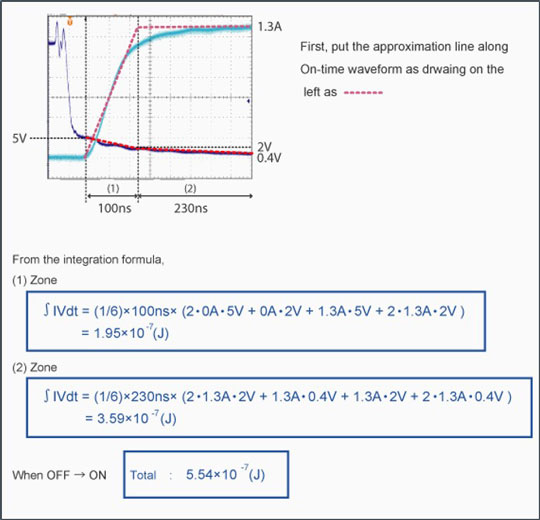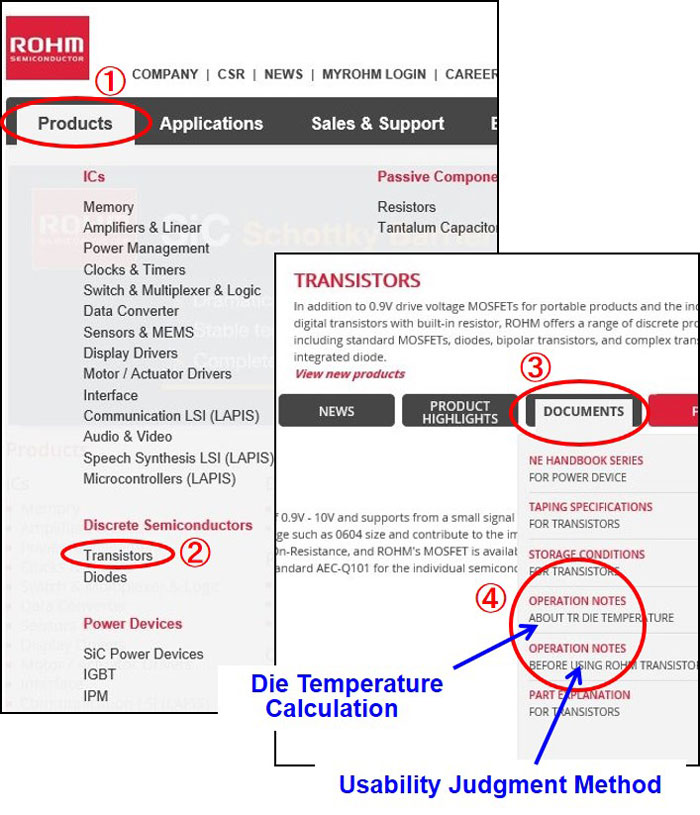Technical Information Site of Power Supply Design

• Basic Knowledge
• AC/DC
• Design Method of PWM AC/DC Flyback Converters
• Designing Isolated Flyback Converter Circuits: Selecting Critical Components – MOSFET related - 1

2016.06.09 AC/DC

# Designing Isolated Flyback Converter Circuits: Selecting Critical Components – MOSFET related - 1

Design Method of PWM AC/DC Flyback Converters

After completing the design of a transformer, we now move on to the switching element. In this section we select a MOSFET Q1 and construct a related circuit.

In the first step, we select a MOSFET Q1 based upon a switching voltage and current. This will be explained in this article [Selecting Critical Components – MOSFET related - 1].After that, we determine the circuit (diode D4, resistors R5 and R6) that adjusts the gate drive for the MOSFET. Subsequently, we also determine the current sense resistor R8 that is necessary for current limiting and slope compensation. These items will be explained in the [Selecting Critical Components – MOSFET related -2] that follows.

We now describe the operation of the circuit in this part. The signal from the OUT (PWM output) pin of the IC is adjusted in the elements D4, R5, and R6 to ensure that the MOSFET Q1 will operate properly, and that the MOSFET gate is driven. The MOSFET Q1 turns on and off the high voltage that was input to the primary side of the transformer T1 and rectified, and transfers the resulting energy to the secondary side. Turning Q1 on causes the Ids to flow. However, because the current cannot be allowed to flow in unlimited amounts, the resistor R8 is used to sense and limit the current. See the overall circuit described in the section [Designing Isolated Flyback Converter Circuits].

Selecting a MOSFET Q1

The first thing that must be understood is that because selection of MOSFET is difficult to perform based exclusively on a priori calculations, empirical formulas are needed. Ultimately, an actual model is used to verify the extent of derating that is needed, and to determine the MOSFET that must be selected.

For the selection of a MOSFET, the following basic items must be examined:

• Maximum drain–source voltage (Vds）
• Peak current
• Loss due to on-resistance (Ron)
• The maximum allowable power dissipation for the package (Pd)

If empirical formulas are not available, and one cannot make a selection without an adequate basis, we examine both Vds and Ids as follows:

(1) Vds (max)

The value of Vds (max) can be determined from the following equation:

Vds(max) = Vin(max)+VOR+Vspike
=264V×1.41＋(12V＋1V)×30/6＋Vspike＝437V＋Vspike*

VOR：VO＝Vout＋VF multiplied by the transformer winding ratio Np:Ns

See「Transformer Design (Calculating numerical values)
Vin(max): The peak value of the corresponding maximum AC voltage (264V×√2）
Vspike: Spike voltage

*Because Vspike is difficult to calculate, in this example, assuming that a snubber circuit will be added and based on an empirical formula, the value of Vspike is assumed to be 400V or less.

(2) Ids

As general rule, for Ids select a value approximately equal to Ippk×2. From
[Transformer Design (Calculating numerical values)], we obtain Ippk＝2.32A.

Or, Ids＝2.32×2＝4.64A

From this, we select a MOSFET with a value of Vds (max) roughly equal to 800V and an Ids value of 5A. In the example circuit, Rohm’s R8005ANX（800V, 5A） is selected. Also, the on-resistance of this MOSFET is 1.6Ω, and the package is TO-220F.

Subsequently, using this MOSFET in an actual circuit we measure the values for Vds and Ids, and the amount of heat generated to verify whether the level of derating achieved is good enough. Because the lower the input voltage the longer is the MOSFET on-time and the greater is the heat generation due to on-resistance Ron losses, especially in the case of worldwide input (AC85V to AC264V), particular attention is required. A heat sink must be provided as necessary to provide adequate heat dissipation.

Some MOSFET manufacturers provide losses determination methods and a method for estimating such losses. An example is given below for your reference.By clicking on items beginning with ① indicated as COPY on the home page screen, you can view pages that provide device temperature calculation methods and criteria by which you can assess whether the device is adoption worthy. This information may be useful for checking out a device in an actual device.

This concludes the selection of a MOSFET. The gate drive adjustment circuit and current detection resistors will be explained in [Selecting Critical Components – MOSFET related -2].

#### Key Points:

・Select a switching transistor (MOSFET) principally based on the drain-source voltage,
the peak current, loss due to on-resistance,and allowable power dissipation for the package.

・Because it is difficult to make a rigorous selection based exclusively on paper and pencil calculations,
you need empirical formulas and measurements on actual devices.